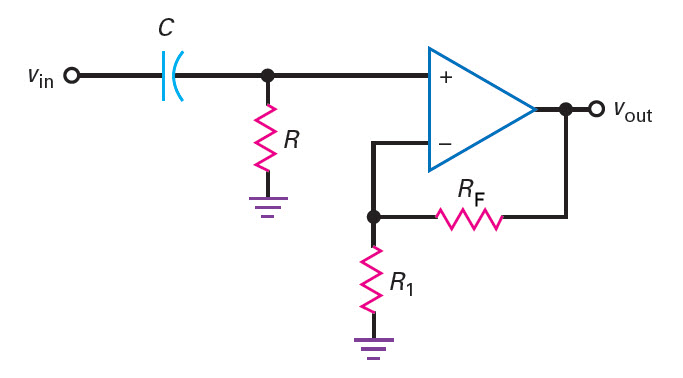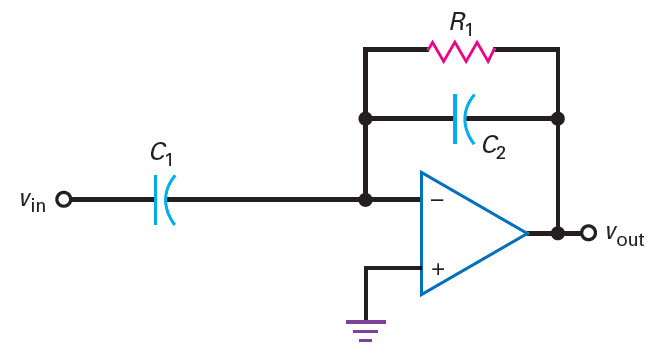# First Order Active High Pass Filter Calculator Online

The following are online calculator for first order active high pass filter using non-inverting and inverting configuration of the operational amplifier.In case of non-inverting configuration based active high pass filter, the cutoff frequency(fc) of the HPF and the capacitor value(C) is provided by the user. The calculator calculates the value for the resistor R1 for the desired passband gain (AF) and feedback resistor RF provided by the user. In case of inverting configuration based active high pass filter, the resistor R value is calculated from the cutoff frequency(fc)and capacitor value provided by the user. The feedback resistor value is calculated from the passband gain (AF) and the already calculated resistor R value

Non-Inverting Active 1st HPF Calculator
Inputs:

Formula Used:
$$R = \frac{1}{2 \pi f_c C}\, \\ R_1 = \frac{R_F}{A_F - 1}$$

Theoretical Results:Inverting Active 1st HPF Calculator
Inputs:

Formula Used:
$$R_1 = \frac{1}{2 \pi f_c C_2}\, \\ C_1 = -A_F C_2$$

Theoretical Results:## Notes on First Order Active High Pass Filter Calculator

• Cutoff frequency
The cutoff frequency of the non-inverting active HPF is given by,

$f_c=\frac{1}{2 \pi R C}$

The cutoff frequency of the inverting active HPF is given by,

$f_c=\frac{1}{2 \pi R_1 C_2}$
• Passband gain
The passband gain of the non-inverting HPF is given by,

$$A_F = 1 + \frac{R_f}{R_1}$$

The passband gain of the inverting HPF is given by,

$$A_F = - \frac{C_1}{C_2}$$

•  Capacitor Values
For best result select the capacitor C value in the range 0.001uF and 0.1uF.